# NCERT Solutions for Class 7 Maths Chapter 2 Fractions and Decimals Ex 2.2

NCERT Solutions for Class 7 Maths Chapter 2 Fractions and Decimals Ex 2.2

### NCERT Solutions for Class 7 Maths Chapter 2 Fractions and Decimals Ex 2.2

NCERT Solutions for Class 7 Maths Chapter 2 Fractions and Decimals Exercise 2.2
Ex 2.2 Class 7 Maths Question 1.
Which of the drawings (a) to (d) show.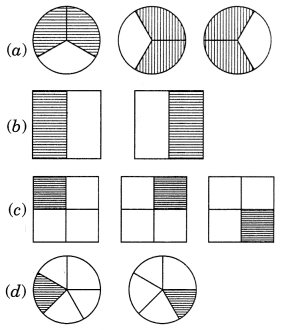Solution:Ex 2.2 Class 7 Maths Question 2.
Some pictures (a) to (c) are given below. Tell which of them show: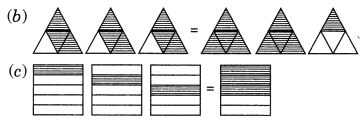Solution:Ex 2.2 Class 7 Maths Question 3.
Multiply and reduce to lowest form and convert into a mixed fraction: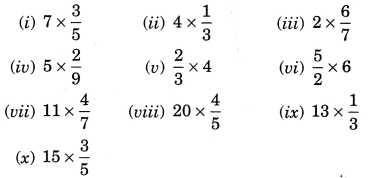Solution: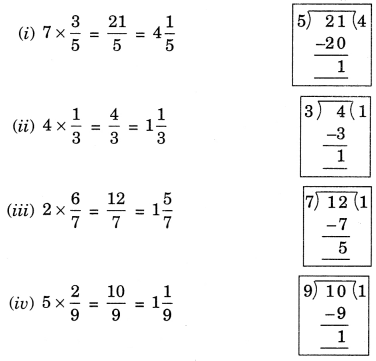Ex 2.2 Class 7 Maths Question 4.
(i) 1/2 of the circles in box (a)
(ii) 2/3 of the circles in box (b)
(iii) 3/5 of the circles in box (c)Solution: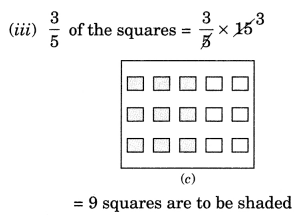Ex 2.2 Class 7 Maths Question 5.
Find:Solution: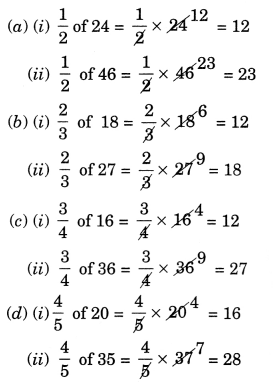Ex 2.2 Class 7 Maths Question 6.
Multiply and express as a mixed fraction.Solution:Ex 2.2 Class 7 Maths Question 7.
Find:Solution:Ex 2.2 Class 7 Maths Question 8.
Vidya and Pratap went for a picnic. Their mother gave them a water bottle that contained 5 litres of water. Vidya consumed 2/5 of the water. Pratap consumed the remaining water.
(i) How much water did Vidya drink?
(ii) What fraction of the total quantity of water did Pratap drink?
Solution:
(i) Water consumed by Vidya = 2/5 of 5 litres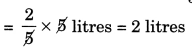Water consumed by Patap
= 5 litres – 2 litres = 3 litres
(ii) Fraction of water consumed by Pratap
3/5 litres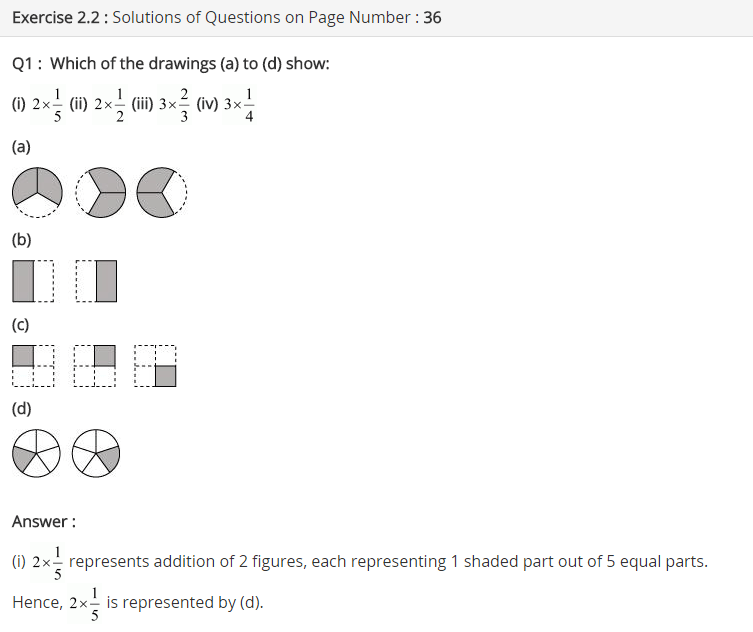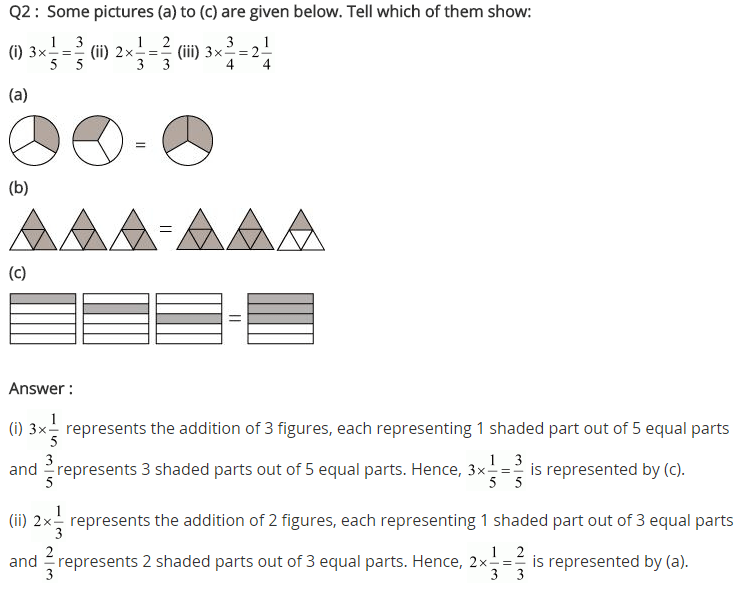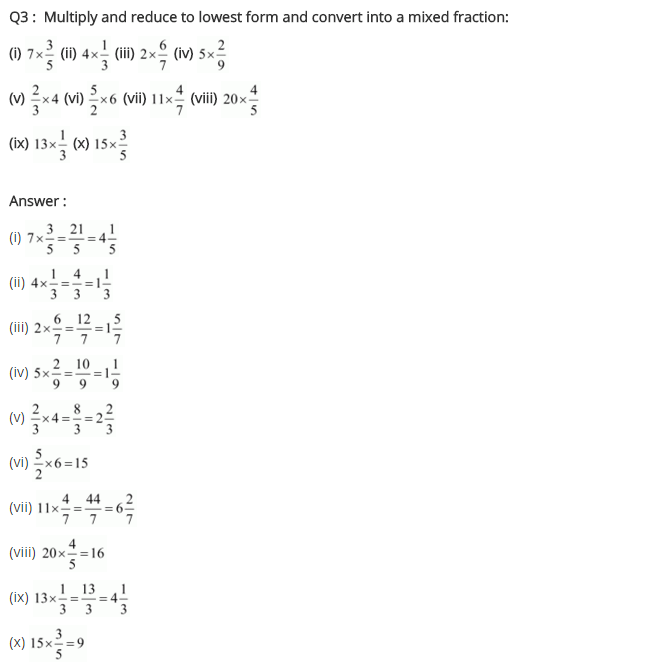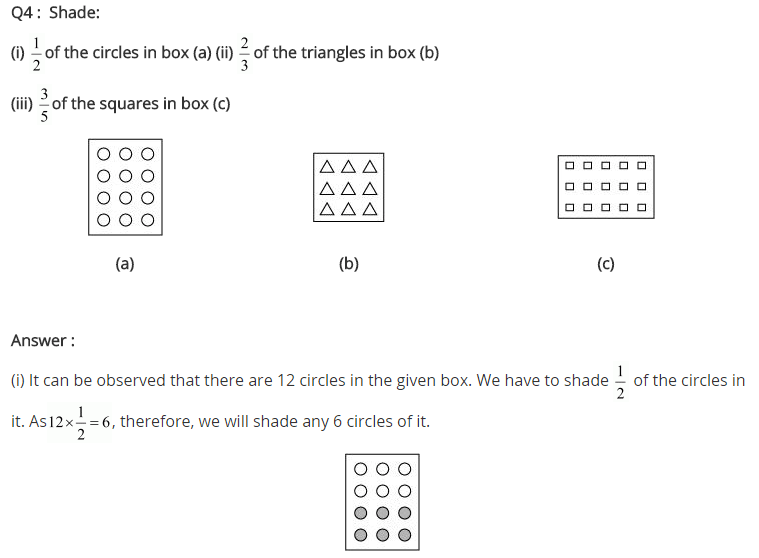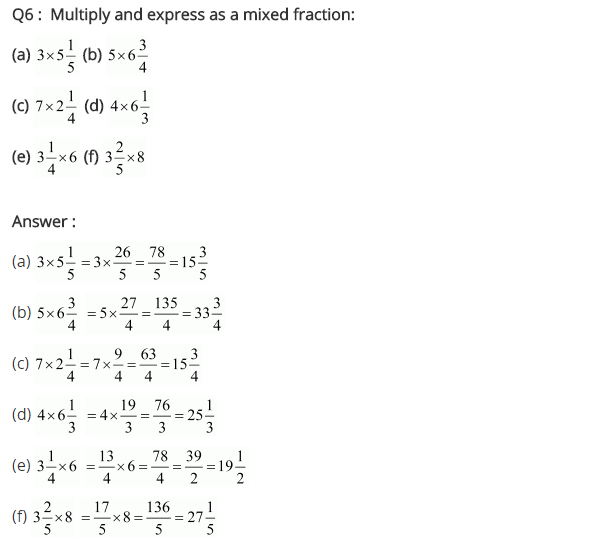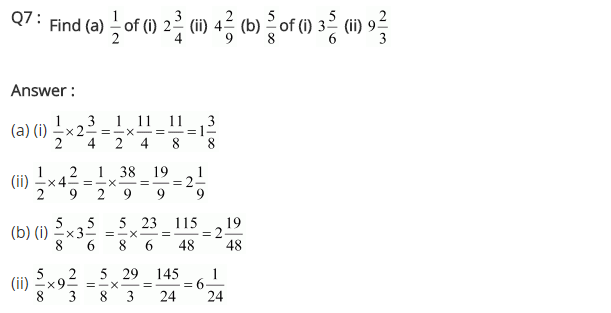## SabDekho

The Complete Educational Website